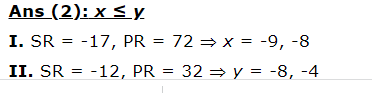# Math: Quadratic Equation Questions Quiz-7

Hello Aspirants, As we all know that Quadratic Equation Questions is a important part of quantitative aptitude section for every competitive exams.Different kind of Quadratic Equation Questions asked in every competitative exam.So here, In this article we will provide Quadratic Equation Quiz.These Quadratic Equation Questions are important for Bank, SSC, SEBI, NABARD, RBI, LIC, and Other state exams. You can attempt these questions & boost your preparation for your examination.
In the Banking exams Quadratic Equation Questions asked in the Prelims as well as Mains exam.There are 5 Quadratic Equation Questions asked in the Prelims exam (Bank).You want to score more in the Quadratic Equation section then you should practice more and more Quadratic Equation. .😃😃

Join Here – Now you can join our Telegram Channel to get new PDF and other Study Material!

Direction (Qs.1-5): Study the following table carefully and answer the questions given below:

1➤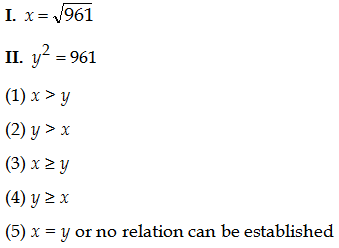=>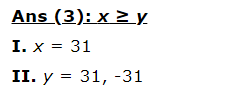2➤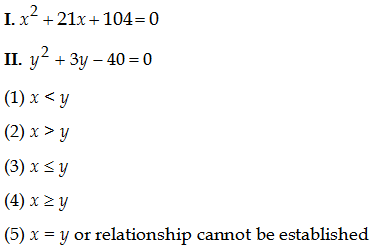=>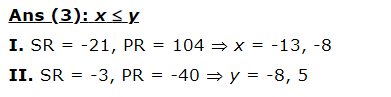3➤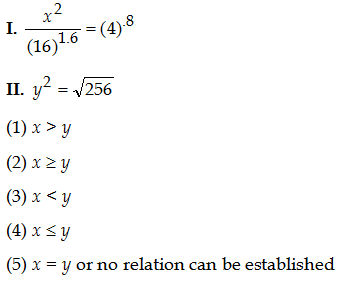=>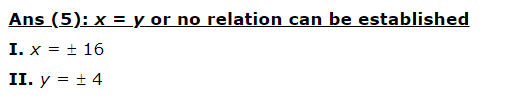4➤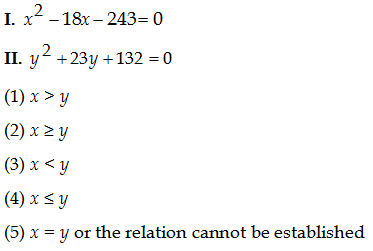=>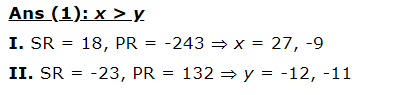5➤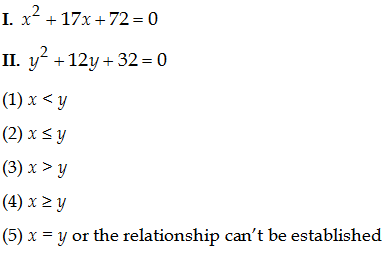=>